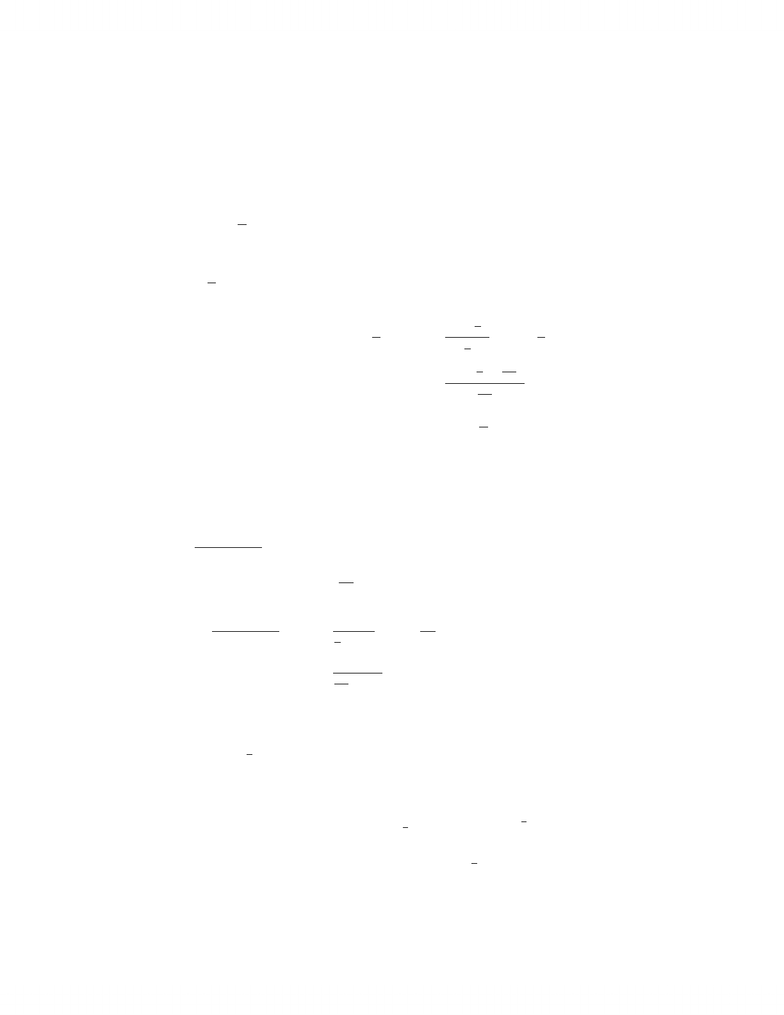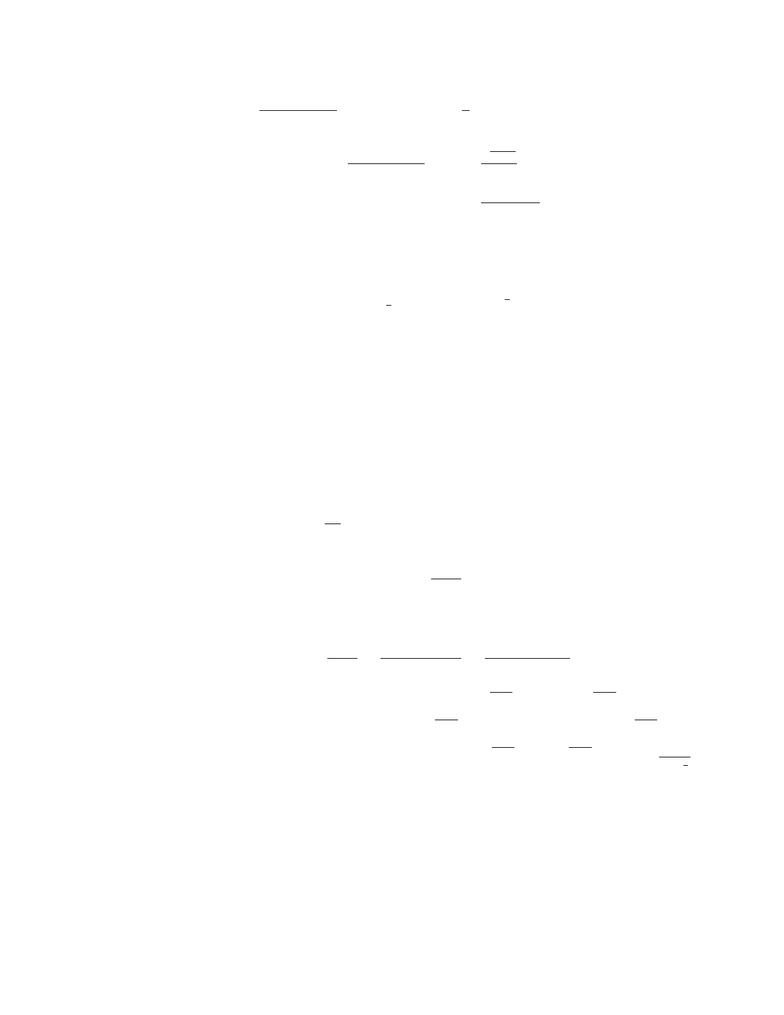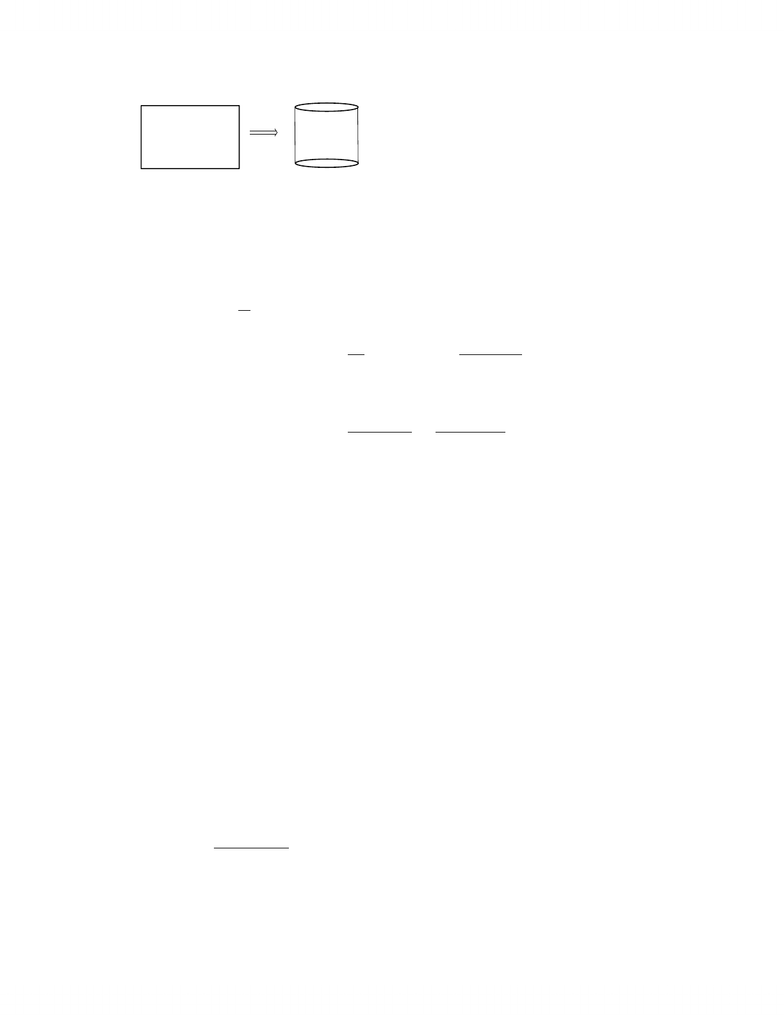Study Guides (390,000)
CA (150,000)
Western (10,000)
CALC (100)
Quiz

# Calculus 1000A/B Study Guide - Quiz Guide: Polynomial Long Division, Asymptote

Department
Calculus
Course Code
CALC 1000A/B
Professor
Chris Brandl
Study Guide
Quiz

This preview shows pages 1-2. to view the full 8 pages of the document.MATH 127 Fall 2012
Assignment 8 Solutions
1. Evaluate the following limits.
(a) lim
x→∞
xsin 1
x
This is of the type ·0 so we have an indeterminate product. We will manipulate
xsin 1
xso that we can apply L’Hopital’s rule.
lim
x→∞
xsin 1
x= lim
x→∞
sin 1
x
1
x=0
0
= lim
x→∞
cos 1
x1
x2
1
x2
= lim
x→∞ cos 1
x
= cos (0)
= 1
(b) lim
x→∞
x2
ln (x) + ex
This is a limit of the type
so we use L’Hopital’s rule.
lim
x→∞
x2
ln (x) + ex= lim
x→∞
2x
1
x+ex=
so we apply L0Hopital0s rule again
= lim
x→∞
2
1
x2+ex
= 0
(c) lim
x0+(x+ex)3
x
This is of the type 1so we have an indeterminate power. Notice that
lim
x0+(x+ex)3
x= lim
x0+eln(x+ex)3
x
= lim
x0+e3
xln(x+ex)
1

Only pages 1-2 are available for preview. Some parts have been intentionally blurred.Consider lim
x0+
3 ln (x+ex)
x. This of the type 0
0so we apply L’Hopital’s rule.
lim
x0+
3 ln (x+ex)
x= lim
x0+
31+ex
x+ex
1
= lim
x0+
3(1 + ex)
x+ex
= 6
Thus,
lim
x0+(x+ex)3
x= lim
x0+eln(x+ex)3
x=e6
2. A closed box is to be constructed so that its base length is three times the base width
and is to have a volume of 450 cm3. Find the dimensions of the box so that it has the
smallest possible surface area.
Let wbe the width, hbe the height, and lbe the length of the box. We then have
that the length of the box is l= 3w. So then the volume is given by (3w)wh = 450
and the surface area is given by s= 2(3w2) + 2(3w)h+ 2wh = 6w2+ 8wh. From the
volume equation we get h=150
w2. Substituting this into the surface area equation we
get
s= 6w2+1200
w
Diﬀerentiating we get
s0= 12w1200
w2=12w31200
w3=12(w3100)
w3
From this we see that the critical points are w= 0,3
100 but w=3
100 is the only
reasonable value. We have that if 0 < w < 3
100 then s0<0 and if w > 3
100 then
s0>0. From the ﬁrst derivative test we see that w=3
100, l= 3 3
100, and h=150
1002
3
give the dimensions of the box that has the smallest surface area.
3. A rectangular sheet of metal with a perimeter of 36 cm and dimensions xand yis to
be rolled into a cylinder. What dimensions of the sheet of metal would maximize the
volume of the cylinder?
2

Unlock to view full version

Only pages 1-2 are available for preview. Some parts have been intentionally blurred.x
yy
We want to maximize v=πr2ysubject to the constraint that 2x+ 2y= 36. Isolating
for yin the perimeter equation we get y= 18 x. Substituting this into the volume
equation we get v=πr2(18 x). We want an equation for the volume in terms of
a single variable. Notice that the circumference of the cylinder is given by 2πr =x
which gives r=x
2π. Substituting this into the volume equation we get
v=πx
2π2(18 x) = 18x2x3
4π
Diﬀerentiating we get
v0=36x3x2
4π=x(36 3x)
4π
We see that the critical points are x= 0,12 but since xis the length of the rectangular
sheet we get that x= 12 is the only reasonable value. We have that if 0 <x<12 then
v0>0 and if x > 12 then v0<0. Thus by the ﬁrst derivative test we get that x= 12
maximizes the volume. Hence, the dimensions should be x= 12 and y= 6.
4. For the following functions
(i) Find the domain, intercepts, and asymptotes of the function.
(ii) Find the critical points, intervals where fis increasing and decreasing, and the
local extrema.
(iii) Find the intervals of concavity and inﬂection points of f.
(iv) Sketch the graph.
(a) f(x) = 2xex2
(b) f(x) = x23x4
3x
3